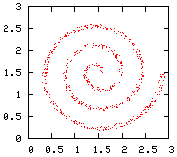## Kernel PCA for novelty detection

This website accompanies the article "Kernel PCA for Novelty Detection" (Pattern Recognition, 2007). The site provides matlab-code of the algorithm and the two-dimensional point distributions used in the article. Please, cite this article if you use any of the material presented here for any publication.

### Matlab code demonstrating the algorithm

In kernel PCA for novelty detection, the reconstruction error in feature space is used as a measure for novelty. The following matlab code demonstrates the reconstruction error for a given 2-D point distribution and shows the resulting decision boundary enclosing all data points.

Download code:
Download and unzip the file kpca.zip [8 KB]. The zip archive contains three m-files: `kpcabound.m`, `recerr.m`, and `kernel.m`. The last two are helper functions for the demo program `kpcabound`. `kpcabound` has as input the data set, the kernel parameter (here, sigma of a Gaussian function), the number of eigenvectors to be extracted, and the number of points outside the decision boundary.

Test code:
For the following test, you need the file `ring-line-square.dat` from the data-distribution archive below. In matlab, type

`A = load('ring-line-square.dat');`
`kpcabound(A,0.4,40,0)`

These commands should result in the following figure:If you look closely at the resulting decision boundaries, you may find a small difference from the curves published in the article. This difference results from the coarser grid used in the matlab code to compute the reconstruction-error surface (the grid is coarser to increase computation speed).

### Two-dimensional synthetic distributions

The following archive contains five distributions: square, square-noise, ring-line-square, spiral, and sine-noise. Their generation is described in the above mentioned article.

Attributes: Two columns contain the (x,y)-coordinates of each data point.Download: testdist.zip [28 KB]

Install: type `unzip testdist.zip` (in Windows use, e.g., Winzip or GnuWin UnZip)
Unzipping will extract five files: `square.dat`, `square-noise.dat`, `ring-line-square.dat`, `spiral.dat`, and `sine-noise.dat`.

15 Apr 2007